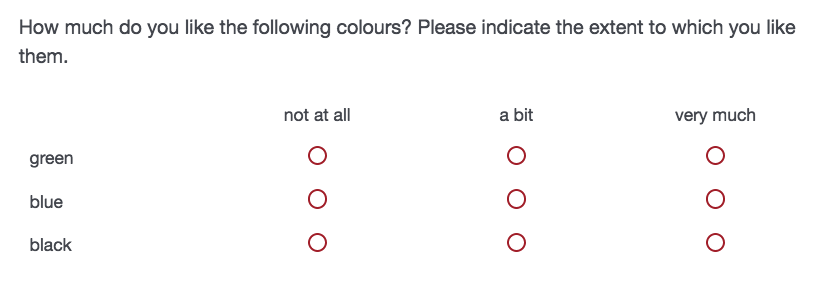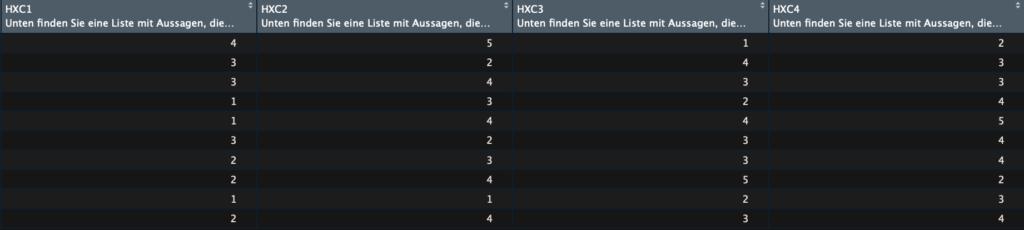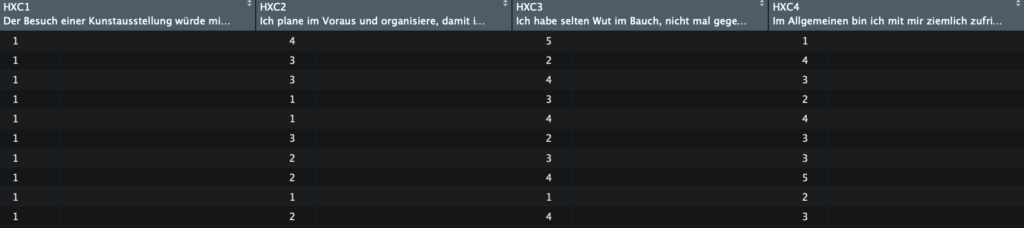# Import and tidy SPSS files generated by Qualtrics

## Overview

Importing SPSS files (ending in .sav) from Qualtrics into R is relatively straightforward. You can, for instance, use the package `haven`:

``df <- read_spss("~/myDataFromQualtrics.sav")``

A strength of this package is that it also imports the labels of the items (i.e., the exact wording of the question; see example below).

One thing that I find terribly unpractical is that Qualtrics adds a lot of extra information to some types of questions that make it difficult to recognize the actual content of questions. For example, if you have a matrix question in which you ask participants to indicate how much they like different colours (see Figure 1), then the item labels will repeat the text before the question for every single item of the matrix. That is, in your dataframe in R, the first item would be called “How much do you like the following colours? Please indicate the extent to which you like them.–green”. These long names make it difficult to find the information that you are actually interested in (here: “green”).Figure 1. Screenshot showing a typical matrix question; the introductory text will be repeated for each item.

A solution to get rid of this unnecessary extra information is to use a function that Stefan Borer, Jasper Ginn, and I wrote to import .csv files in the package qualtRics.

### A function to tidy item labels from Qualtrics

If you want to get rid of the repetitive and unnecessary text in front of your actual item content, simply copy the following code into R (or R Studio) and run it. The default option is to also remove html tags. (If you want to keep html code, set stripHTML = FALSE).

``````# start function
tidy_label <- function(file_name,
stripHTML = TRUE) {

item_labels <- sjlabelled::get_label(file_name)

# Remove html
if(stripHTML) {
pattern <- "</?\\w+((\\s+\\w+(\\s*=\\s*(?:\".*?\"|'.*?'|[^'\">\\s]+))?)+\\s*|\\s*)/?>" # nolint
item_labels <- gsub(pattern, "\\4", item_labels)
}

# Remove text in front of matrix questions, return subquestion:
# If it matches one of ".?!" followed by "-", take subsequent part
subquestions <- stringr::str_match(item_labels, ".*[:punct:]\\s*-(.*)")[,2]

# Else if subquestion returns NA, use whole string
subquestions[is.na(subquestions)] <- unlist(item_labels[is.na(subquestions)])

# Remaining NAs default to 'empty string'
subquestions[is.na(subquestions)] <- ""

file_name <- sjlabelled::set_label(file_name, unlist(subquestions))
return(file_name)
}``````

After you have run the code above, you can use the function on your data and get clean item labels:

``my_clean_df <- tidy_label(df)``

Here’s a comparison of how your data look like in the R Studio viewer:Figure 2. Before cleaning; the same intro text appears in each item that was included in the matrix.Figure 3. After cleaning item labels; the actual content of the items is visible.